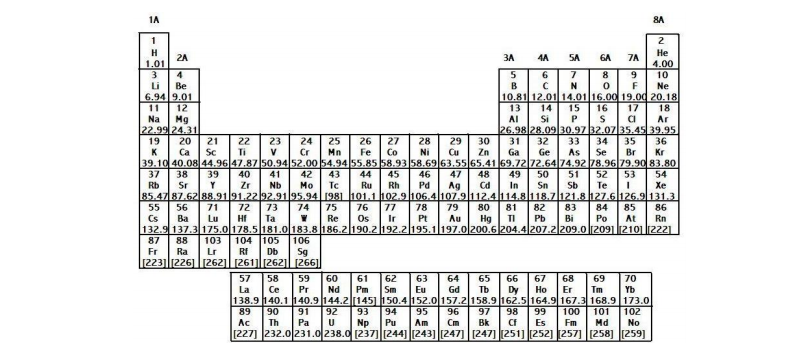# Problem: Write the formula and the phase for the following elements.a) Chlorine  b) Argon  c) Bromine  d) Zinc

###### FREE Expert Solution
89% (214 ratings)
###### Problem Details

Write the formula and the phase for the following elements.

a) Chlorine

b) Argon

c) Bromine

d) ZincFrequently Asked Questions

What scientific concept do you need to know in order to solve this problem?

Our tutors have indicated that to solve this problem you will need to apply the Periodic Table: Phases concept. You can view video lessons to learn Periodic Table: Phases. Or if you need more Periodic Table: Phases practice, you can also practice Periodic Table: Phases practice problems.

What is the difficulty of this problem?

Our tutors rated the difficulty ofWrite the formula and the phase for the following elements.a...as low difficulty.

How long does this problem take to solve?

Our expert Chemistry tutor, Jules took 3 minutes and 48 seconds to solve this problem. You can follow their steps in the video explanation above.

What professor is this problem relevant for?

Based on our data, we think this problem is relevant for Professor Biberdorf's class at TEXAS.